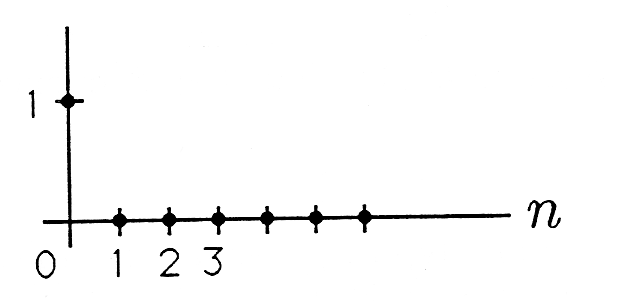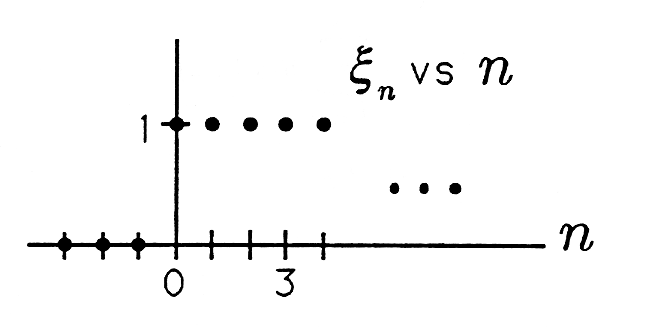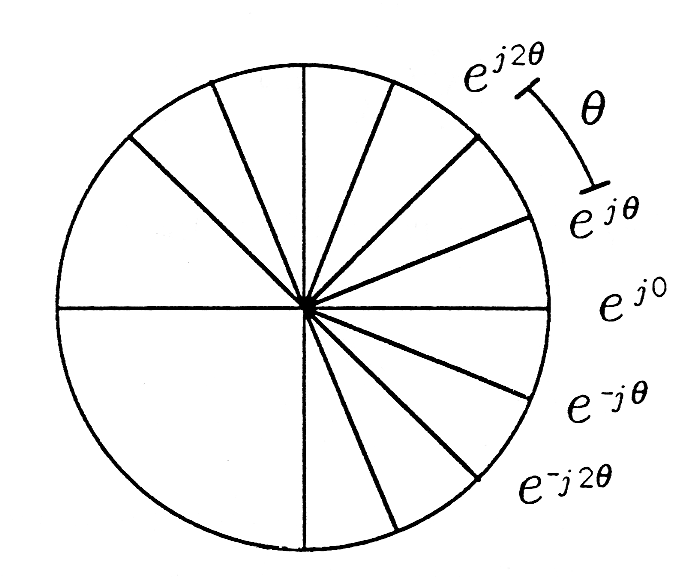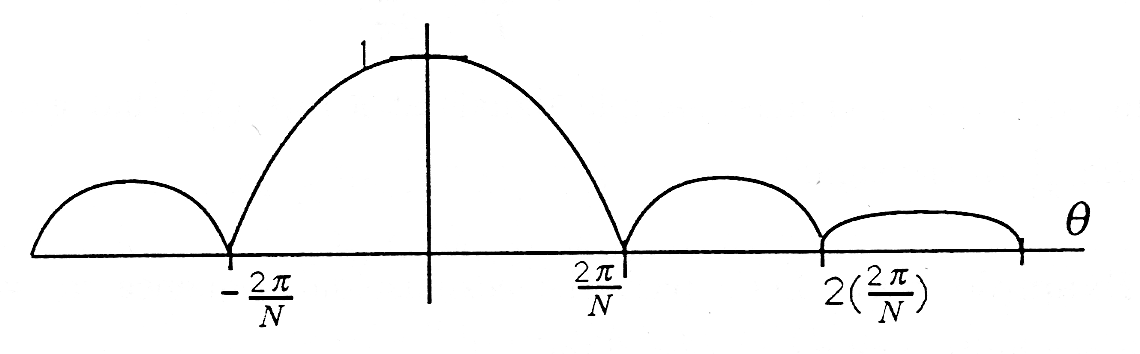# 7.5 Filtering: test sequences

 Page 1 / 1
This module is part of the collection, A First Course in Electrical and Computer Engineering . The LaTeX source files for this collection were created using an optical character recognition technology, and because of this process there may be more errors than usual. Please contact us if you discover any errors.

When we design a filter, we design it for a purpose. For example, a moving average filter is often designed to pass relatively constant data whileaveraging out relatively variable data. In an effort to clarify the behavior of a filter, we typically analyze its response to a standard set of test signals. Wewill call the impulse , the step , and the complex exponential the standard test signals.

Unit Pulse Sequence. The unit pulse sequence is the sequence

${u}_{n}={\delta }_{n}=1,n=00,n\ne 0.$

This sequence, illustrated in Figure 1 , consists of all zeros except for a single one at $n=0$ . If the unit pulse sequence is passed through a moving average filter (whether finite or not), then the output is called the unit pulse response :

${h}_{n}=\sum k=0\infty {w}_{k}{\delta }_{n-k}={w}_{n}.$Unit Pulse Sequence

(Note that ${\delta }_{n-k}=0$ unless $n=k.$ ) So the unit pulse sequence may be used to read out the weights of a moving average filter. It is common practice to use ${w}_{k}$ (the ${k}^{th}$ weight) and ${h}_{k}$ (the ${k}^{th}$ impulse response) interchangeably.

Unit Step Sequence. The unit step sequence is the sequence

${u}_{n}={\xi }_{n}=1,n\ge 00,n<0.$

This sequence is illustrated in Figure 2 . When this sequence is applied to a moving average filter, the result is the unit step response

${g}_{n}=\sum k=0n{w}_{k}=\sum k=0n{h}_{k}.$

The unit step response is just the sequence of partial sums of the unit pulse response.Unit Step Sequence

Complex Exponential Sequence. The complex exponential sequence is the sequence

${u}_{k}={e}^{jk\theta },k=0,±1,±2,...$

This sequence, illustrated in Figure 3 , is a “discrete-time phasor” that “ratchets” counterclockwise (CCW) as $k$ moves to $k+1$ and clockwise (CW) as $k$ moves to $k-1$ . Each time the phasor ratchets, it turns out an angle of $\theta$ . Why should such a sequence be a useful test sequence? There are two reasons.Discrete-Time Phasor

(i) ${e}^{jk\theta }$ represents (or codes) $cosk\theta$ . The real part of the sequence ${e}^{jk\theta }$ is the cosinusoidal sequence $cosk\theta$ :

$Re\left[{e}^{jk\theta }\right]=cosk\theta .$

Therefore the discrete-time phasor ${e}^{jk\theta }$ represents (or codes) $cosk\theta$ in the same way that the continuous-time phasor ${e}^{j\omega t}$ codes $cos\omega t$ . If the moving average filter

${x}_{n}=\sum k=0\infty {h}_{k}{u}_{n-k}$

has real coefficients, we can get the response to a cosinusoidal sequence by taking the real part of the following sum:

${x}_{n}=\sum k=0\infty {h}_{k}cos\left(n-k\right)\theta =Re\left[\sum k=0\infty {h}_{k}{e}^{j\left(n-k\right)\theta }\right]=Re\left[{e}^{jn\theta }\sum k=0\infty {h}_{k}{e}^{-jk\theta }\right].$

In this formula, the sum

$\sum k=0\infty {h}_{k}{e}^{-jk\theta }$

is called the complex frequency response of the filter and is given the symbol

$H\left({e}^{j\theta }\right)=\sum k=0\infty {h}_{k}{e}^{-jk\theta }.$

This complex frequency response is just a complex number, with a magnitude $|H\left({e}^{j\theta }\right)|$ and a phase arg $H\left({e}^{j\theta }\right)$ . Therefore the output of the moving average filter is

${x}_{n}=Re\left[{e}^{jn\theta }H\left({e}^{j\theta }\right)\right]=Re\left[{e}^{jn\theta }|H\left({e}^{j\theta }\right)|{e}^{jargH\left({e}^{j\theta }\right)}\right]=|H\left({e}^{j\theta }\right)|cos\left[n\theta +argH\left({e}^{j\theta }\right)\right].$

This remarkable result says that the output is also cosinusoidal, but its amplitude is $|H\left({e}^{j\theta }\right)|$ rather than 1, and its phase is $argH\left({e}^{j\theta }\right)$ rather than 0. In the examples to follow, we will show that the complex “gain” $H\left({e}^{j\theta }\right)$ can be highly selective in $\theta$ , meaning that cosines of some angular frequencies are passed with little attenuation while cosines of other frequencies are dramatically attenuated. By choosing the filter coefficients, we can design the frequency selectivity we would like to have.

(ii) ${\mathit{\text{e}}}^{jk\theta }$ is a sampled data version of ${e}^{j\omega t}$ . The discrete-time phasor ${e}^{jk\theta }$ can be produced physically by sampling the continuous-time phasor ${e}^{j\omega t}$ at the periodic sampling instants ${t}_{k}=kT$ :

${e}^{jk\theta }={e}^{j\omega t}{|}_{t=kT}={e}^{j\omega kT}\theta =\omega T.$

The dimensions of $\theta$ are radians, the dimensions of $\omega$ are radians/second, and the dimensions of $T$ are seconds. We call $T$ the sampling interval and $\frac{1}{T}$ the sampling rate or sampling frequency. If the original angular frequency of thephasor ${e}^{j\omega t}$ is increased to $\omega +m\left(\frac{2\pi }{T}\right)$ , then the discrete-time phasor remains ${e}^{jk\theta }$ :

${e}^{j\left[\omega +m\left(2\pi /T\right)\right]t}{|}_{t=kT}={e}^{j\left(\omega kT+km2\pi \right)}={e}^{jk\theta }.$

This means that all continuous-time phasors of the form ${e}^{j\left[\omega +m\left(2\pi /T\right)\right]t}$ “hide under the same alias” when viewed through the sampling operation. Thatis, the sampled-data phasor cannot distinguish the frequency $\omega$ from the frequency $\omega +m\frac{2\pi }{T}$ . In your subsequent courses you will study aliasing in more detail and study the Nyquist rule for sampling:

$T\le \frac{2\pi }{\Omega };\frac{1}{T}\ge \frac{\Omega }{2\pi }$

This rule says that you must sample signals at a rate $\left(\frac{1}{T}\right)$ that exceeds the bandwidth $\frac{\Omega }{2\pi }$ of the signal.

Let's pass the cosinusoidal sequence ${u}_{k}=$ cos $k\theta$ through the finite moving average filter

${x}_{n}=\sum k=0N-1{h}_{k}{u}_{n-k}{h}_{k}=\frac{1}{N}k=0,1,...,N-1.$

We know from our previous result that the output is

${x}_{n}=|H\left({e}^{j\theta }\right)|cos\left[n\theta +argH\left({e}^{j\theta }\right)\right].$

The complex frequency response for this example is

$H\left({e}^{j\theta }\right)=\sum k=0N-1\frac{1}{N}{e}^{-jk\theta }=\frac{1}{N}\frac{1-{e}^{-jN\theta }}{1-{e}^{-j\theta }}$

(Do you see your old friend, the finite sum formula, at work?) Let's try to manipulate the result into a more elegant form:

$H\left({e}^{j\theta }\right)=\frac{1}{N}\frac{{e}^{-j\left(N/2\right)\theta }\left[{e}^{j\left(N/2\right)\theta }-{e}^{-j\left(N/2\right)\theta }\right]}{{e}^{-j\left(\theta /2\right)}\left[{e}^{j\left(\theta /2\right)}-{e}^{-j\left(\theta /2\right)}\right]}=\frac{1}{N}{e}^{-j\left[\left(N-1\right)/2\right]\theta }\frac{sin\left(\frac{N}{2}\theta \right)}{sin\left(\frac{1}{2}\theta \right)}.$

The magnitude of the function $H\left({e}^{j\theta }\right)$ is

$|H\left({e}^{j\theta }\right)|=\frac{1}{N}\text{,}|\frac{sin\left(\frac{N}{2}\theta \right)}{sin\left(\frac{1}{2}\theta \right)}|.$

At $\theta =0$ , corresponding to a “DC phasor,” $H\left({e}^{j\theta }\right)$ equals 1; at $\theta =\frac{2\pi }{N}$ $|H\left({e}^{j\theta }\right)=0|$ . The magnitude of the complex frequency response is plotted in Figure 4 .Frequency Selectivity of a Moving Average Filter

This result shows that the moving average filter is frequency selective, passing low frequencies with gain near 1 and high frequencies with gain near 0.

#### Questions & Answers

Is there any normative that regulates the use of silver nanoparticles?
Damian Reply
what king of growth are you checking .?
Renato
What fields keep nano created devices from performing or assimulating ? Magnetic fields ? Are do they assimilate ?
Stoney Reply
why we need to study biomolecules, molecular biology in nanotechnology?
Adin Reply
?
Kyle
yes I'm doing my masters in nanotechnology, we are being studying all these domains as well..
Adin
why?
Adin
what school?
Kyle
biomolecules are e building blocks of every organics and inorganic materials.
Joe
anyone know any internet site where one can find nanotechnology papers?
Damian Reply
research.net
kanaga
sciencedirect big data base
Ernesto
Introduction about quantum dots in nanotechnology
Praveena Reply
what does nano mean?
Anassong Reply
nano basically means 10^(-9). nanometer is a unit to measure length.
Bharti
do you think it's worthwhile in the long term to study the effects and possibilities of nanotechnology on viral treatment?
Damian Reply
absolutely yes
Daniel
how to know photocatalytic properties of tio2 nanoparticles...what to do now
Akash Reply
it is a goid question and i want to know the answer as well
Maciej
characteristics of micro business
Abigail
for teaching engĺish at school how nano technology help us
Anassong
Do somebody tell me a best nano engineering book for beginners?
s. Reply
there is no specific books for beginners but there is book called principle of nanotechnology
NANO
what is fullerene does it is used to make bukky balls
Devang Reply
are you nano engineer ?
s.
fullerene is a bucky ball aka Carbon 60 molecule. It was name by the architect Fuller. He design the geodesic dome. it resembles a soccer ball.
Tarell
what is the actual application of fullerenes nowadays?
Damian
That is a great question Damian. best way to answer that question is to Google it. there are hundreds of applications for buck minister fullerenes, from medical to aerospace. you can also find plenty of research papers that will give you great detail on the potential applications of fullerenes.
Tarell
what is the Synthesis, properties,and applications of carbon nano chemistry
Abhijith Reply
Mostly, they use nano carbon for electronics and for materials to be strengthened.
Virgil
is Bucky paper clear?
CYNTHIA
carbon nanotubes has various application in fuel cells membrane, current research on cancer drug,and in electronics MEMS and NEMS etc
NANO
so some one know about replacing silicon atom with phosphorous in semiconductors device?
s. Reply
Yeah, it is a pain to say the least. You basically have to heat the substarte up to around 1000 degrees celcius then pass phosphene gas over top of it, which is explosive and toxic by the way, under very low pressure.
Harper
Do you know which machine is used to that process?
s.
how to fabricate graphene ink ?
SUYASH Reply
for screen printed electrodes ?
SUYASH
What is lattice structure?
s. Reply
of graphene you mean?
Ebrahim
or in general
Ebrahim
in general
s.
Graphene has a hexagonal structure
tahir
On having this app for quite a bit time, Haven't realised there's a chat room in it.
Cied
what is biological synthesis of nanoparticles
Sanket Reply
how did you get the value of 2000N.What calculations are needed to arrive at it
Smarajit Reply
Privacy Information Security Software Version 1.1a
Good
Got questions? Join the online conversation and get instant answers!
Jobilize.com Reply

### Read also:

#### Get the best Algebra and trigonometry course in your pocket!

Source:  OpenStax, A first course in electrical and computer engineering. OpenStax CNX. Sep 14, 2009 Download for free at http://cnx.org/content/col10685/1.2
Google Play and the Google Play logo are trademarks of Google Inc.

Notification Switch

Would you like to follow the 'A first course in electrical and computer engineering' conversation and receive update notifications?ByBy